# AI For Trading:Dependent Labels Exercise (114)

## Overlapping Labels

In this exercise, we're going to simulate the issue we encounter when training machine learning models using targets that are returns that overlap in time. The core issue is that these labels are correlated and in fact redundant. We'll see what impact this has on our machine learning algorithm, and we'll have the opportunity to implement some of the solutions to the problem that we described in the lectures, and will later encounter in the project.

import numpy as np
import pandas as pd
import matplotlib.pyplot as plt
from tqdm import tqdm_notebook as tqdm

%matplotlib inline
plt.style.use('ggplot')
plt.rcParams['figure.figsize'] = (14, 8)

Let's load up a small dataset of artificial "toy" data that we can play with. The columns in these data are A, B, C and D. We will use the E column as targets.

df = pd.read_csv('dependent_labels_dataset.csv', index_col = 0)
df.head()
A B C D E
t
1.0 0.780067 -1.139543 0.298152 0.412867 3
2.0 0.521061 -0.680001 0.148710 0.461035 3
3.0 0.425336 -0.620970 0.099168 0.409145 3
4.0 0.696707 -0.666513 0.099526 0.477195 3
5.0 -0.059698 -0.220574 0.249784 0.465186 3
df.plot()
<matplotlib.axes._subplots.AxesSubplot at 0x7f51b57e7fd0>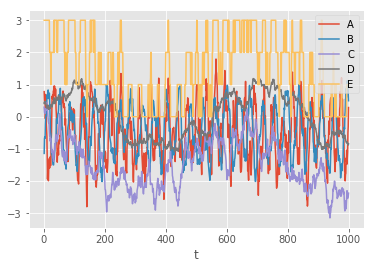df.shape
(1000, 5)

## Create redundancy in the data

In order to illustrate the effect of redundancy in the data, we are going to deliberately create an extreme version of this condition by duplicating each row in the data 5 times, using the function create_redundant_data below.

num_duplicates = 5
def create_redundant_data(df, num_duplicates):
"""
From the existing dataset, create a new dataset in which every original row is exactly duplicated num_duplicates times.
Re-order this new dataset according to the order of the original index.

Parameters
----------
df : pandas DataFrame
The original dataset.

Returns
-------
redundant_df : pandas DataFrame
The new, redundant dataset.
"""
redundant_df = df.copy()

for i in range(num_duplicates - 1):
redundant_df = redundant_df.append(df)

redundant_df.sort_index(axis=0, level='t', inplace=True)

return redundant_df
redundant_df = create_redundant_data(df, num_duplicates)
redundant_df.shape
(5000, 5)

Create a function for scoring the model.

def model_score(m, X_train, y_train, X_valid, y_valid):
'''
Take in the model and training and validation datasets, and return the training accuracy score, validation
accuracy score, and out-of-bag score. Furthermore, print each of these results.

Parameters
----------
m : RandomForestClassifier instance
The trained model.

X_train : pandas DataFrame
The training features.

y_train : pandas Series
The training labels.

X_valid : pandas DataFrame
The validation features.

y_valid : pandas Series
The validation labels.

Returns
-------
train_score : float
The mean training accuracy.

valid_score : float
The mean validation accuracy.

oob_score : float
The out-of-bag score.

'''

# TODO: Implement
train_score = m.score(X_train, y_train)
valid_score = m.score(X_valid, y_valid)
oob_score = m.oob_score_

return train_score, valid_score, oob_score

## Split data into train, valid and test sets

def make_splits(df, features, target, split_valid=0.20, split_test=0.20):
temp = df.dropna()

X = temp[features].copy()
y = temp[target].copy()

train_end = int(X.shape*(1-split_valid-split_test))
valid_end = train_end + int(X.shape*split_valid)

X_train, X_valid, X_test = X.iloc[:train_end,], X.iloc[(train_end+1):valid_end,], X.iloc[(valid_end+1):]
y_train, y_valid, y_test = y.iloc[:train_end,], y.iloc[(train_end+1):valid_end,], y.iloc[(valid_end+1):]

return X, X_train, X_valid, X_test, y_train, y_valid, y_test
features = ['A', 'B', 'C', 'D']
X, X_train, X_valid, X_test, y_train, y_valid, y_test = make_splits(
redundant_df,
features,
'E'
)

## Train one tree, take a look at it

from sklearn.ensemble import RandomForestClassifier
def instantiate_and_fit_one_tree(X_train, y_train):
"""
Instantiate a single decision tree and fit it on the training data. Return the fitted classifier.

Parameters
----------
X_train : pandas DataFrame
The training features.

y_train : pandas Series
The training labels.

Returns
-------
clf : DecisionTreeClassifier
The fitted classifier instance.
"""

clf = RandomForestClassifier(
n_estimators=1,
max_depth=3,
max_features=None,
bootstrap=True,
criterion='entropy',
random_state=0
)

clf.fit(X_train.values, y_train)
return clf
clf = instantiate_and_fit_one_tree(X_train, y_train)
! pip install graphviz
Collecting graphviz
Installing collected packages: graphviz
Successfully installed graphviz-0.11.1
import graphviz
from sklearn.tree import export_graphviz

def export_graph(classifier, feature_names):
"""
First, export the dot data from the fitted classifier. Then, create a graphviz Source object from the dot data,
and return it.

Parameters
----------
classifier : DecisionTreeClassifier
The single decision tree you created and fit above.

Returns
-------
graph : graphviz Source object
The Source object created with the graph information.
"""

dot_data = export_graphviz(
clf.estimators_,
out_file=None,
feature_names=features,
filled=True, rounded=True,
special_characters=True,
rotate=False
)
graph = graphviz.Source(dot_data);
return graph
# display the single decision tree graph in the notebook
graph = export_graph(clf, features)
graph

## Redundant labels

Let's see what happens when we train a full random forest on these redundant data.

def instantiate_and_fit_a_rf(n_estimators, X_train, y_train, min_samples_leaf=5):
"""
Instantiate a random forest classifier and fit it on the training data. Return the fitted classifier.
Make sure you use bootstrapping and calculate an out-of-bag score. Set random_state equal to an integer so that
when you fit the model again you get the same result.

Parameters
----------
n_estimators : int
The number of trees.

X_train : pandas DataFrame
The training features.

y_train : pandas Series
The training labels.

Returns
-------
clf : RandomForestClassifier
The fitted classifier instance.
"""

clf = RandomForestClassifier(
n_estimators=n_estimators,
max_features='sqrt',
min_samples_leaf=min_samples_leaf,
bootstrap=True,
oob_score=True,
n_jobs=-1,
criterion='entropy',
verbose=0,
random_state=0
)
clf.fit(X_train, y_train)
return clf
train_score = []
valid_score = []
oob_score = []
tree_sizes = [10, 50, 100, 250]

for trees in tqdm(tree_sizes):
clf_red = instantiate_and_fit_a_rf(trees, X_train, y_train)

tr, va, oob = model_score(clf_red, X_train, y_train, X_valid, y_valid)
train_score.append(tr); valid_score.append(va); oob_score.append(oob)


Failed to display Jupyter Widget of type HBox.

If you're reading this message in the Jupyter Notebook or JupyterLab Notebook, it may mean that the widgets JavaScript is still loading. If this message persists, it likely means that the widgets JavaScript library is either not installed or not enabled. See the Jupyter Widgets Documentation for setup instructions.

If you're reading this message in another frontend (for example, a static rendering on GitHub or NBViewer), it may mean that your frontend doesn't currently support widgets.

/opt/conda/lib/python3.6/site-packages/sklearn/ensemble/forest.py:453: UserWarning: Some inputs do not have OOB scores. This probably means too few trees were used to compute any reliable oob estimates.
warn("Some inputs do not have OOB scores. "
/opt/conda/lib/python3.6/site-packages/sklearn/ensemble/forest.py:458: RuntimeWarning: invalid value encountered in true_divide
predictions[k].sum(axis=1)[:, np.newaxis])
def plot_results(tree_sizes, train_score, oob_score, valid_score, title, y_range):
plt.plot(tree_sizes, train_score, 'xb-');
plt.plot(tree_sizes, oob_score, 'xg-');
plt.plot(tree_sizes, valid_score, 'xr-');
plt.title(title);
plt.xlabel('Number of Trees');
plt.ylabel('Accuracy')
plt.legend(['train','oob', 'valid'])
plt.ylim(y_range, y_range);
y_range = []
y_range.append(0.45)
y_range.append(1.005)
plot_results(tree_sizes, train_score, oob_score, valid_score, 'Random Forest with Redundant Data (accuracy): train, validation, oob', y_range)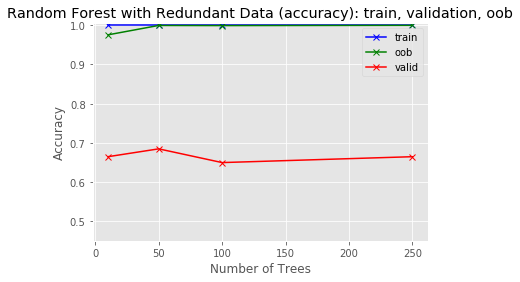What you can see from this run is that the OOB score virtually tracks the training score because the OOB data contains the same information as the training data. The validation score, calculated on unseen data, is much lower.

## Solution #1: use every 5th row

In this section, implement the first solution to the redundancy issue by sub-sampling every 5th row of the data.

def create_subsampled_dataset(num_duplicates, X_train, y_train):
"""
Create the sub-sampled dataset according to the first solution proposed to the problem of overlapping labels.

Parameters
----------
num_duplicates : int
The number of duplications made earlier.

X_train : pandas DataFrame
The training features.

y_train : pandas Series
The training labels.

Returns
-------
X_train_sub : pandas DataFrame
The training features, subsampled.

y_train_sub : pandas Series
The training labels, subsampled.
"""

X_train_sub = X_train[::5]
y_train_sub = y_train[::5]

return X_train_sub, y_train_sub
X_train_sub, y_train_sub = create_subsampled_dataset(5, X_train, y_train)
train_score = []
valid_score = []
oob_score = []
tree_sizes = [10, 50, 100, 250]

for trees in tqdm(tree_sizes):
clf_sub = instantiate_and_fit_a_rf(trees, X_train_sub, y_train_sub)

tr, va, oob = model_score(clf_sub, X_train_sub, y_train_sub, X_valid, y_valid)
train_score.append(tr); valid_score.append(va); oob_score.append(oob)


Failed to display Jupyter Widget of type HBox.

If you're reading this message in the Jupyter Notebook or JupyterLab Notebook, it may mean that the widgets JavaScript is still loading. If this message persists, it likely means that the widgets JavaScript library is either not installed or not enabled. See the Jupyter Widgets Documentation for setup instructions.

If you're reading this message in another frontend (for example, a static rendering on GitHub or NBViewer), it may mean that your frontend doesn't currently support widgets.

/opt/conda/lib/python3.6/site-packages/sklearn/ensemble/forest.py:453: UserWarning: Some inputs do not have OOB scores. This probably means too few trees were used to compute any reliable oob estimates.
warn("Some inputs do not have OOB scores. "
/opt/conda/lib/python3.6/site-packages/sklearn/ensemble/forest.py:458: RuntimeWarning: invalid value encountered in true_divide
predictions[k].sum(axis=1)[:, np.newaxis])
plot_results(tree_sizes, train_score, oob_score, valid_score, 'Random Forest on Redundant Data, with Subsampling (accuracy): train, validation, oob', y_range)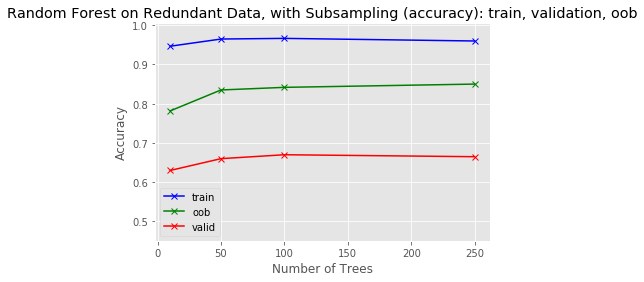In the case of our artificial dataset, this completely removes the redundancy.

## Solution #2: reduce bag size

In this section, implement the second solution we proposed—to use BaggingClassifier to randomly draw a smaller fraction of the original dataset's rows when creating each tree's dataset.

from sklearn.ensemble import BaggingClassifier
from sklearn.tree import DecisionTreeClassifier
base_clf = DecisionTreeClassifier(
criterion='entropy',
max_features='sqrt',
min_samples_leaf=5
)
def instantiate_and_fit_a_BaggingClassifier(n_estimators, base_estimator, X_train, y_train, max_samples = 0.2):
"""
Instantiate a Bagging Classifier and fit it on the training data. Return the fitted classifier.
Make sure you use bootstrapping and calculate an out-of-bag score. Set random_state equal to an integer so that
when you fit the model again you get the same result.

Parameters
----------
n_estimators : int
The number of trees.

base_estimator : DecisionTreeClassifier
The base estimator of the BaggingClassifier.

max_samples : float
The percentage of the number of original rows to draw for each bag.

X_train : pandas DataFrame
The training features.

y_train : pandas Series
The training labels.

Returns
-------
clf : BaggingClassifier
The fitted classifier instance.
"""

clf = BaggingClassifier(
base_estimator = base_estimator,
n_estimators = n_estimators,
max_samples = max_samples,
bootstrap=True,
oob_score=True,
n_jobs=-1,
verbose=0,
random_state=0
)
clf.fit(X_train, y_train)
return clf

train_score = []
valid_score = []
oob_score = []
tree_sizes = [10, 50, 100, 250]

for trees in tqdm(tree_sizes):
clf_bag = instantiate_and_fit_a_BaggingClassifier(trees, base_clf, X_train, y_train)

tr, va, oob = model_score(clf_bag, X_train, y_train, X_valid, y_valid)
train_score.append(tr); valid_score.append(va); oob_score.append(oob)

Failed to display Jupyter Widget of type HBox.

If you're reading this message in the Jupyter Notebook or JupyterLab Notebook, it may mean that the widgets JavaScript is still loading. If this message persists, it likely means that the widgets JavaScript library is either not installed or not enabled. See the Jupyter Widgets Documentation for setup instructions.

If you're reading this message in another frontend (for example, a static rendering on GitHub or NBViewer), it may mean that your frontend doesn't currently support widgets.

plot_results(tree_sizes, train_score, oob_score, valid_score, 'Random Forest on Redundant Data, with Reduced Bag Size (accuracy): train, validation, oob', y_range)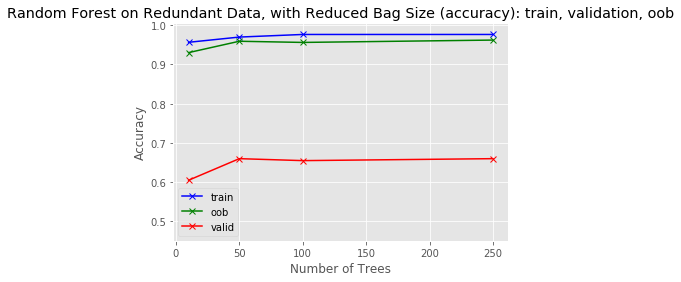As you can see, in the case of this small artificial dataset, this helped but didn't fully resolve the problem. The OOB score still vastly overestimates the validation score.

## Solution #3: bagged non-overlapping labels

Finally, let's look at the last solution we proposed to this problem. We'll do this one for you for this small exercise, but you'll have the opportunity to work on it yourself later. We will fit separate models on each non-redundant subset of data, and then ensemble them together.

from sklearn.ensemble import VotingClassifier
from sklearn.base import clone
from sklearn.preprocessing import LabelEncoder
from sklearn.utils.validation import has_fit_parameter, check_is_fitted
from sklearn.utils.metaestimators import _BaseComposition
from sklearn.utils import Bunch
class NoOverlapVoter(VotingClassifier):
def __init__(self, base_estimator, overlap_increment=5):
self.est_list = []
for i in range(overlap_increment):
self.est_list.append(('clf'+str(i), clone(base_estimator)))
self.overlap_increment = overlap_increment
super().__init__(
self.est_list,
voting='soft'
)

@property
def oob_score_(self):
oob = 0
for clf in self.estimators_:
oob = oob + clf.oob_score_
return oob / len(self.estimators_)

def fit(self, X, y, sample_weight=None):
names, clfs = zip(*self.estimators)
self._validate_names(names)

self.le_ = LabelEncoder().fit(y)
self.classes_ = self.le_.classes_
self.estimators_ = []

transformed_y = self.le_.transform(y)

self.estimators_ = []
for i in range(self.overlap_increment):
self.estimators_.append(
clfs[i].fit(X[i::self.overlap_increment], transformed_y[i::self.overlap_increment])
)

self.named_estimators_ = Bunch(**dict())
for k, e in zip(self.estimators, self.estimators_):
self.named_estimators_[k] = e
return self
train_score = []
valid_score = []
oob_score = []
tree_sizes = [10, 50, 100, 250]

for trees in tqdm(tree_sizes):
clf = RandomForestClassifier(
n_estimators=trees,
max_features='sqrt',
min_samples_leaf=5,
bootstrap=True,
oob_score=True,
n_jobs=-1,
criterion='entropy',
verbose=0,
random_state=0
)

clf_nov = NoOverlapVoter(clf)
clf_nov.fit(X_train.reset_index()[['A','B','C','D']], y_train)
t, v, o = model_score(clf_nov, X_train, y_train, X_valid, y_valid)
train_score.append(t); valid_score.append(v); oob_score.append(o)

Failed to display Jupyter Widget of type HBox.

If you're reading this message in the Jupyter Notebook or JupyterLab Notebook, it may mean that the widgets JavaScript is still loading. If this message persists, it likely means that the widgets JavaScript library is either not installed or not enabled. See the Jupyter Widgets Documentation for setup instructions.

If you're reading this message in another frontend (for example, a static rendering on GitHub or NBViewer), it may mean that your frontend doesn't currently support widgets.

/opt/conda/lib/python3.6/site-packages/sklearn/ensemble/forest.py:453: UserWarning: Some inputs do not have OOB scores. This probably means too few trees were used to compute any reliable oob estimates.
warn("Some inputs do not have OOB scores. "
/opt/conda/lib/python3.6/site-packages/sklearn/ensemble/forest.py:458: RuntimeWarning: invalid value encountered in true_divide
predictions[k].sum(axis=1)[:, np.newaxis])
/opt/conda/lib/python3.6/site-packages/sklearn/ensemble/forest.py:453: UserWarning: Some inputs do not have OOB scores. This probably means too few trees were used to compute any reliable oob estimates.
warn("Some inputs do not have OOB scores. "
/opt/conda/lib/python3.6/site-packages/sklearn/ensemble/forest.py:458: RuntimeWarning: invalid value encountered in true_divide
predictions[k].sum(axis=1)[:, np.newaxis])
/opt/conda/lib/python3.6/site-packages/sklearn/ensemble/forest.py:453: UserWarning: Some inputs do not have OOB scores. This probably means too few trees were used to compute any reliable oob estimates.
warn("Some inputs do not have OOB scores. "
/opt/conda/lib/python3.6/site-packages/sklearn/ensemble/forest.py:458: RuntimeWarning: invalid value encountered in true_divide
predictions[k].sum(axis=1)[:, np.newaxis])
/opt/conda/lib/python3.6/site-packages/sklearn/ensemble/forest.py:453: UserWarning: Some inputs do not have OOB scores. This probably means too few trees were used to compute any reliable oob estimates.
warn("Some inputs do not have OOB scores. "
/opt/conda/lib/python3.6/site-packages/sklearn/ensemble/forest.py:458: RuntimeWarning: invalid value encountered in true_divide
predictions[k].sum(axis=1)[:, np.newaxis])
/opt/conda/lib/python3.6/site-packages/sklearn/ensemble/forest.py:453: UserWarning: Some inputs do not have OOB scores. This probably means too few trees were used to compute any reliable oob estimates.
warn("Some inputs do not have OOB scores. "
/opt/conda/lib/python3.6/site-packages/sklearn/ensemble/forest.py:458: RuntimeWarning: invalid value encountered in true_divide
predictions[k].sum(axis=1)[:, np.newaxis])
plot_results(tree_sizes, train_score, oob_score, valid_score, 'Random Forest on Redundant Data, with NoOverlapVoter (accuracy): train, validation, oob', y_range)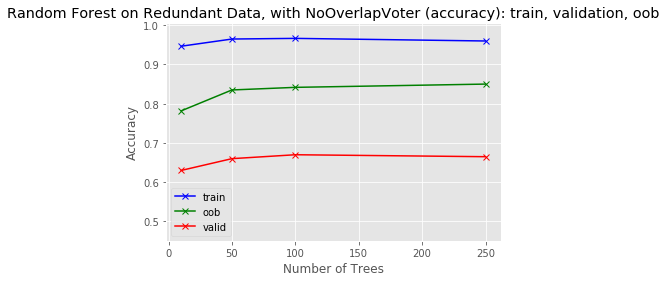So in the case of this artificial dataset, this method performs as well as the first method, but this makes sense because each of the separate classifiers has the exact same information as the others. Combining them together should not yield much performance enhancement. You can then compare this result to the result that you'll see in the project, when this method is applied to real financial data.

## Check the performance on test set

Finally, let's look at the performance of the third method on the held-out test set. For this, we'll train on all of the previous training and validation data.

X, X_train, X_valid, X_test, y_train, y_valid, y_test = make_splits(
redundant_df,
features,
'E',
split_valid = 0
)
clf = RandomForestClassifier(
n_estimators=150,
max_features='sqrt',
min_samples_leaf=5,
bootstrap=True,
oob_score=True,
n_jobs=-1,
criterion='entropy',
verbose=False,
random_state=0
)

clf_nov = NoOverlapVoter(clf)
clf_nov.fit(X_train.reset_index()[['A','B','C','D']], y_train)
t, v, o = model_score(clf_nov, X_train, y_train, X_test, y_test)
train_score.append(t); valid_score.append(v); oob_score.append(o)